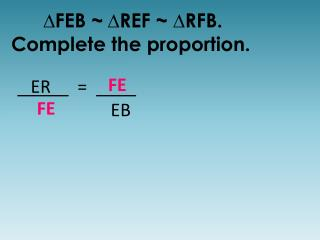# ∆FEB ~ ∆ REF ~ ∆RFB. Complete the proportion. - PowerPoint PPT PresentationDownload Presentation∆FEB ~ ∆ REF ~ ∆RFB. Complete the proportion.

∆FEB ~ ∆ REF ~ ∆RFB. Complete the proportion.Download Presentation## ∆FEB ~ ∆ REF ~ ∆RFB. Complete the proportion.

- - - - - - - - - - - - - - - - - - - - - - - - - - - E N D - - - - - - - - - - - - - - - - - - - - - - - - - - -
##### Presentation Transcript

1. ∆FEB ~∆REF ~ ∆RFB. Complete the proportion. FE FE ER = EB

2. ∆FEB ~∆REF ~ ∆RFB. Complete the proportion. FR FR ER = RB

3. ∆FEB ~∆REF ~ ∆RFB. Complete the proportion. BE BF BF = BR BF = BR EF FR

4. ∆FEB ~∆REF ~ ∆RFB. Complete the proportion. FR BF EF = BE EF = BE ER EF

5. ∆FEB ~∆REF ~ ∆RFB. Complete the proportion. BF BF BE = BR

6. Find the value of x & y. 40 = 45 60 x 40x = 2700 x = 67.5 40 45 55 60 x 40 = 55 100 y 40y = 5500 x = 137.5 y

7. Find the value of x & y. 12 = 18 48 x 12x = 864 x = 72 48 12 60 y 12 = y 60 60 12y = 720 x = 12 18 x

8. ∆EAT ~∆YUM. Find the value of x. T M 5x – 1 6x – 3 18 A Y E U 20 3x + 4 100x – 20 = 54x + 72 46x = 92 x = 2 18 = 20 5x – 1 3x + 4

9. AED SAS~ ∆ABC ~∆______ by _______ C D 150 100 A 90 135 E 90 = 100 . 135 150 13,500 = 13,500 √ B

10. AED AA~ ∆ABC ~∆______ by _______ C D A E B

11. ∆ABC ~∆______ by _______ A D 18 12 22 15 B E C F 15 = 12 . 22.5 18 270 = 270 √ 15 = 14 . 22.5 22 330 ≠ 315 NOT ~ 14 22.5

12. ADB BDC ∆ABC ~∆______ ~∆ _______ A D C B

13. 40 40 50 A = 50 C = _____ABD = ______ CBD = _____ A D C B

14. 47 47 43 WIM = 43 LIM = _____W = ______ L = _____ I W M L

15. WLI ILM ∆WIM ~∆______ ~∆ _______ I W M L

16. RYE YVE ∆RVY ~∆______ ~∆ _______ V E Y R

17. 59 59 31 VYE = 31 EYR = _____V = ______ R = _____ V E Y R

18. A model house is built with a scale of 1’ = 30’. If the width of the model is 7.5’, how wide is the house? 1 = 7.5 . 30 w w = 225’

19. A model house is built with a scale of 1’ = 30’. If the length of the house is 128’, how long is the model? 1 = L . 30 128 30L = 128 L = 4.27’

20. A building that is 73’ tall has a 54.75’ shadow. How long is the shadow of a nearby man who is 6 ½’ tall? 73 = 6.5 . 54.75 x 73x = 355.875 x = 4.875’

21. A 16’tall bulldozer has a 20’ shadow. How tall is the tractor if its shadow is 28’ long? 16 = x . 20 28 20x = 448 x = 22.4’

22. YBD YAB ∆BAD ~∆______ ~∆ _______ B D A Y